# Length Frequency Histograms in ggplot2

Flexibly construct length frequency histograms using ggplot2
Length Frequency
Size
histogram
ggplot2
Author

Derek H. Ogle

Published

Dec 28, 2019

Modified

Mar 19, 2023

Note

The following packages are loaded for use below. I also set the default `ggplot` theme to `theme_bw()` for a classic “black-and-white” plot (rather than the default plot with a gray background).

``````library(ggplot2)
theme_set(theme_bw())``````

# Introduction

A common graphic produced by many fisheries scientists is a length frequency histogram. As it turns out there are a few “tricks” to make a length frequency histogram appear as I expect most fisheries folks would want it to appear – primarily, left-inclusive (i.e., 100 would be in the 100-110 bin and not the 90-100 bin). In this post, I show how to construct a length frequency histograms with `ggplot2` using my preferences.

The data are lengths of Lake Erie Walleye (Sander vitreus) captured during October-November, 2003-2014.1 My primary interest is in the `tl` (total length in mm), `sex`, and `loc` variables2 and I will focus on 2014 (as an example).

• 1 These data are available in the `FSAdata` package and formed many of the examples in Chapter 12 of the Age and Growth of Fishes: Principles and Techniques book.

• ``````data(WalleyeErie2,package="FSAdata")
WE <- dplyr::filter(WalleyeErie2,year==2014)``````

# Basic Length Frequency

Making the histogram (Figure 1) begins by identifying the data frame to use in `data=` and the `tl` variable to use for the `x`-axis as an `aes()`thetic in `ggplot()`. The histogram is then constructed with `geom_histogram()`, which I customize as follows:

• Set the width of the length bins with `binwidth=`.
• By default the bins are centered on breaks created from `binwidth=`. The bins can be changed to begin on these breaks by using `boundary=` set to the desired value of a first break, regardless of whether that break is in the data or not. I use `boundary=0` so that bins will start on breaks that make sense relative to `binwidth=` (e.g., 0, 25, 50, 75, etc.).
• Bins are left-exclusive and right-inclusive by default. Use `closed="left"` to make the bins the desired left-inclusive and right-exclusive.
• The fill color of the bins is set with `fill=` (I prefer a slight gray).
• The outline color of the bins is set with `color=` (defaults to the same as `fill=`; I prefer a dark boundary to make the bins obvious).

The `scale_y_continuous()` and `scale_x_continuous()` are primarily used to provide labels (i.e., `name`s) for the y- and x-axes, respectively. By default, the bins of the histogram will “hover” slightly above the x-axis, which I find annoying. The `expand=` in `scale_y_continuous()` is used to expand the lower limit of the y-axis by a `mult`iple of 0 (thus, not expand the lower-limit) and expand the upper limit of the y-axis by a `mult`iple of 0.05 (thus, the upper-limit will by 5% higher than the tallest bin so that the top frame of the plot will not touch the tallest bin).3

• 3 The resultant plot was assigned to an object so that name must be typed and run to see the plot.

• ``````lenfreq1 <- ggplot(data=WE,aes(x=tl)) +
geom_histogram(binwidth=25,boundary=0,closed="left",
fill="gray80",color="black") +
scale_y_continuous(name="Number of Fish",expand=expansion(mult=c(0,0.05))) +
scale_x_continuous(name="Total Length (mm)")
lenfreq1``````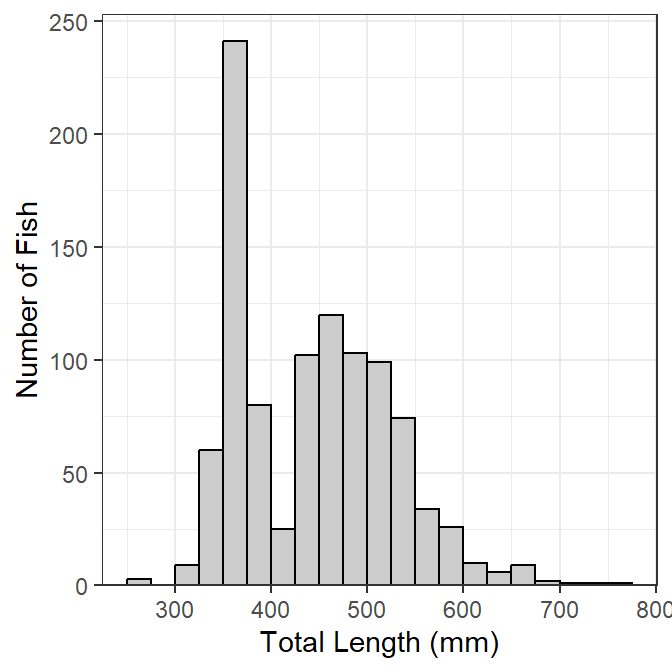Figure 1: Length frequency of histogram of Lake Erie Walleye, 2014.

This base object/plot can be modified by adding (using `+`) to it as demonstrated later.

# Stacked by Other Variable

It may be useful to see the distribution of categories of fish (e.g., sex) within the length frequency bins (Figure 2). To do this, add `fill=` in the `aes()`thetic in `ggplot()` and set it equal to the variable that identifies the separation within each bin (e.g., `sex`). The bins will be stacked by this variable if `position="stack"` in `geom_histogram()`.4 The fill colors for each group can be set in a number of ways, but they are set manually below with `scale_fill_manual()`.

• 4 This is the default and would not need to be explicitly set below).

• ``````lenfreq2 <- ggplot(data=WE,aes(x=tl,fill=sex)) +
geom_histogram(binwidth=25,boundary=0,closed="left",
color="black",position="stack") +
scale_fill_manual(values=c("gray80","gray40")) +
scale_y_continuous(name="Number of Fish",expand=expansion(mult=c(0,0.05))) +
scale_x_continuous(name="Total Length (mm)")
lenfreq2``````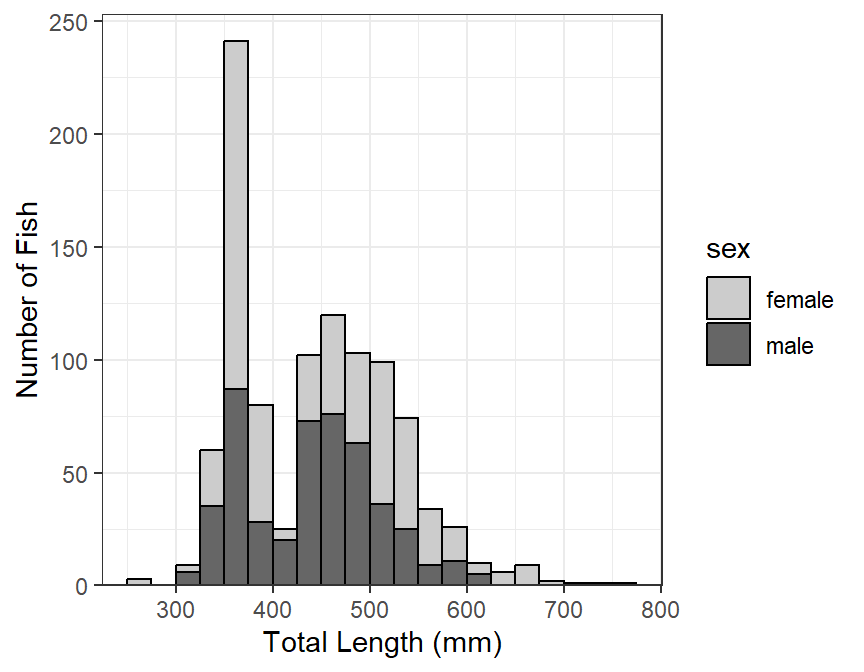Figure 2: Length frequency histogram of Lake Erie Walleye 2014 stacked by sex.

Stacked histograms are difficult to interpret in my opinion. In a future post, I will show how to use empirical density functions to examine distributions among categories. For the time being, see below.

# Separated by Other Variable(s)

A strength of `ggplot2` is that it can easily make the same plot for several different levels of another variable; e.g., separate length frequency histograms by sex (Figure 3). The plot (e.g., `lenfreq` from above) can be separated into different “facets” with `facet_wrap()`, which takes the variable to separate by within `vars()` as the first argument.

``lenfreq1 + facet_wrap(vars(sex))``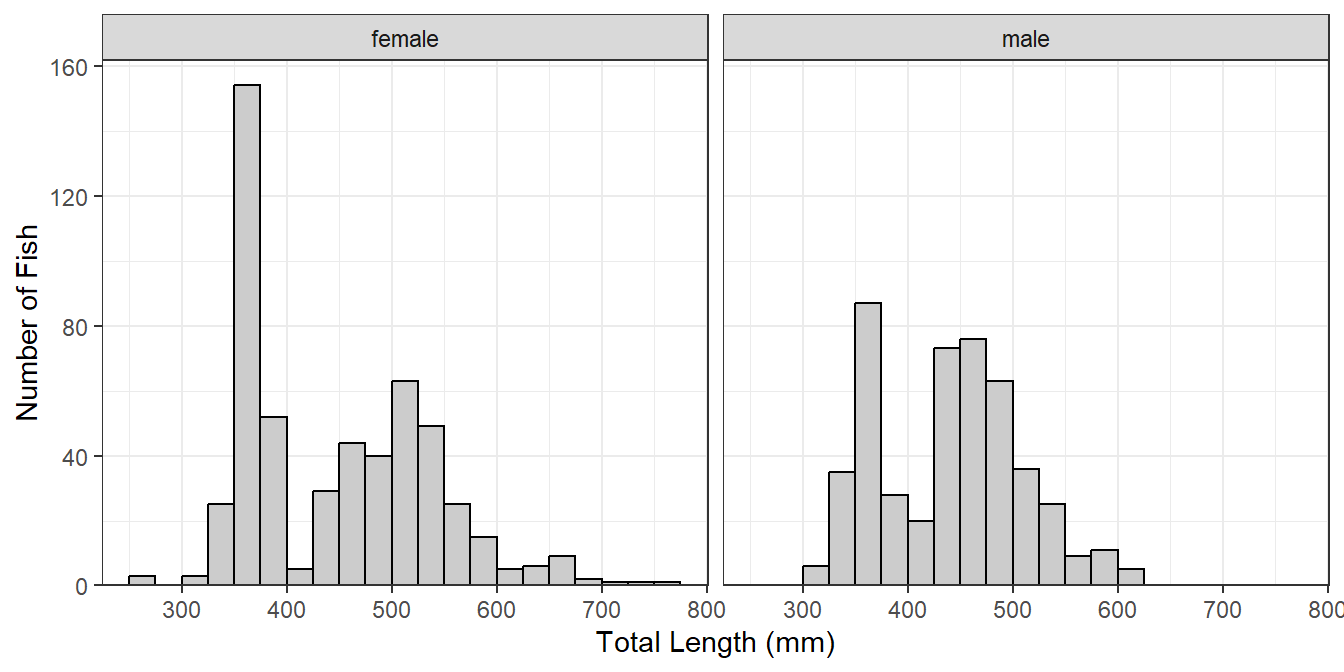Figure 3: Length frequency histogram of Lake Erie Walleye 2014 separated by sex.

If the faceted groups have very different sample sizes then it may be useful to use a potentially different y-axis scale for each facet by including `scales="free_y"` in `facet_wrap()` (Figure 4). Similarly, a potentially different scale can be used for each x-axis with `scales="free_x"` or for both axes with `scales="free"`.

``lenfreq1 + facet_wrap(vars(sex),scales="free_y")``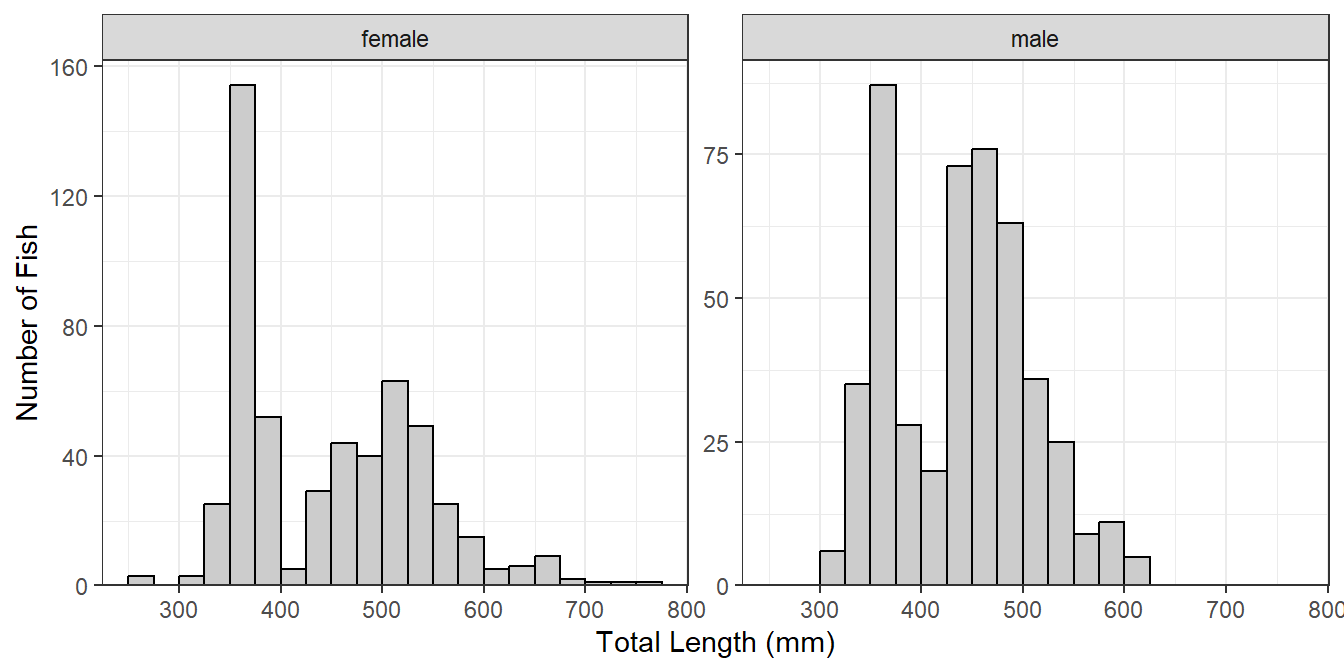Figure 4: Length frequency histogram of Lake Erie Walleye 2014 separated by sex with different frequency scales.

Plots may be faceted over multiple variables with `facet_grid()` (Figure 5), where the variables that identify the rows and variables for a grid of facets are included (within `vars()`) in `rows=` and `cols=`, respectively. Both scales can not be “free” with `facet_grid()`, the scale can only be “free” within a row or column.

``lenfreq1 + facet_grid(rows=vars(loc),cols=vars(sex),scales="free_y")``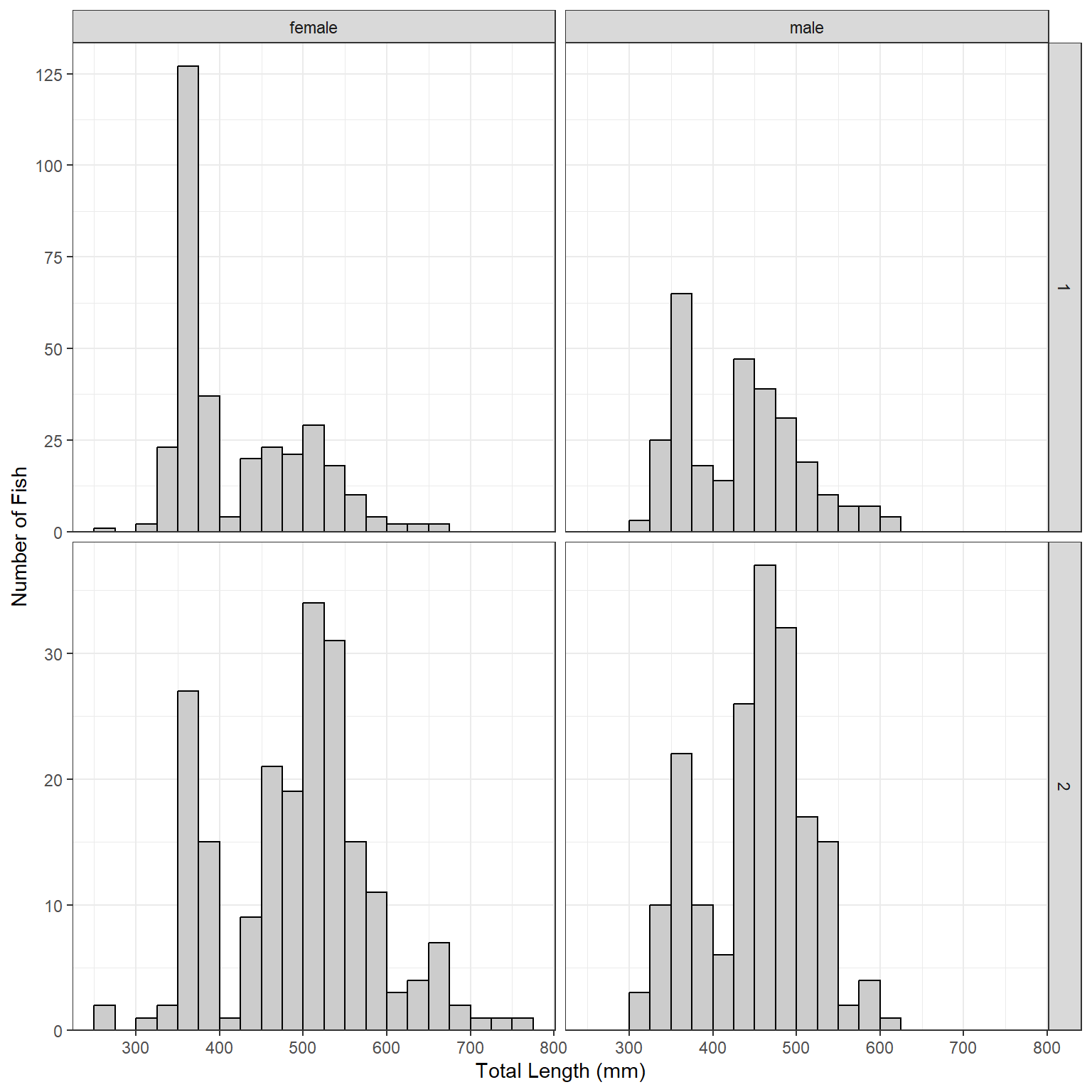Figure 5: Length frequency histogram of Lake Erie Walleye 2014 separated by sex and location of capture with different frequency scales.

## Citation

BibTeX citation:
``````@online{h.ogle2019,
author = {Derek H. Ogle},
title = {Length {Frequency} {Histograms} in Ggplot2},
date = {2019-12-28},
url = {https://fishr-core-team.github.io/fishR//blog/posts/2019-12-28_Length_Frequency_Histograms},
langid = {en}
}
``````